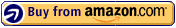This workbook helps your child subtract three-digit numbers with confidence. Children will learn to regroup (borrow) when subtracting, which equips them with the tools to subtract three-digit numbers. Vertical subtraction is included along with patterning and sequencing activities, so children gain a fundamental understanding of subtraction.

Topics Covered in this Book:
-Horizontal subtraction review
-Vertical form subtraction
-Regrouping (borrowing)
-Three-digit minus two-digit subtraction
-Early three- & four-digit subtraction
-Addition & subtraction of three two-digit numbers
-Patterns & sequencing

8 1/2 x 11 inches. paperback. 80 pages. full color.

ISBN: 978-1-933241-52-4

#### US: \$7.95 | CA: \$8.95